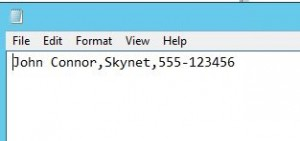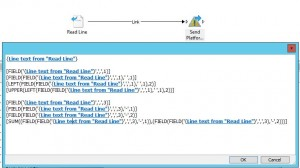# www.contoso.se

### Cloud and Datacenter Management by Anders Bengtsson

Orchestrator support a number of data manipulation functions. These functions can be used to manipulate string and  convert it into a usable form. You can also perform simple arithmetic operations, such as calculating sums and differences, and performing division and multiplication operations. For a complete list of the functions that you can perform see Technet. One nice thing about these data manipulation functions is that you can combine them.

Example, a simple text fileI use a Read Line activity to read the line, and I can then manipulate the data, in this figure I do that in a Send Platform Event activityThe result will be

`John Connor,Skynet,555-123456`
`John Connor`
`John`
`Jo`
`JO`
`555-123456`
`555`
`123456`
`124011`

First line is the text line as in the text file. Then it is
String divided on “,” and showing first part
String divided on “,” and showing first part, then dividing that answer on ” ” and showing first part
String divided on “,” and showing first part, then dividing that answer on ” ” and showing first part, then showing two first letters from the left side
String divided on “,” and showing first part, then dividing that answer on ” ” and showing first part, then showing two first letters from the left side, then UPPER means converting that string to uppercase

String divided on “,” and showing third part
String divided on “,” and showing third part, then dividing that answer on “-” and showing first part
String divided on “,” and showing third part, then dividing that answer on “-” and showing second part
String divided on “,” and showing third part, then dividing that answer on “-” and showing first and second parts, then sum the two numbers

© 2019 www.contoso.se. Theme by Anders Norén.